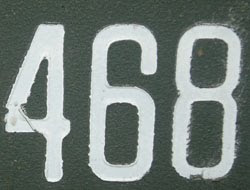## Thursday, October 8, 2009

### 468

468 = 22 x 33 x 13.

468 is the smallest non-trivial number such that its own sum of digits is the same as its square's and its cube's sums of digits: 4 + 6 + 8 = 18; 2 + 1 + 9 + 0 + 2 + 4 = 18; 1 + 0 + 2 + 5 + 0 + 3 + 2 + 3 + 2 = 18.

468 is 3333 in base 5.

468 is the sum of ten consecutive primes: 29 + 31 + 37 + 41 + 43 + 47 + 53 + 59 + 61 + 67 = 468.NGC 468 is a galaxy in the constellation Pisces.

Source: Number Gossip